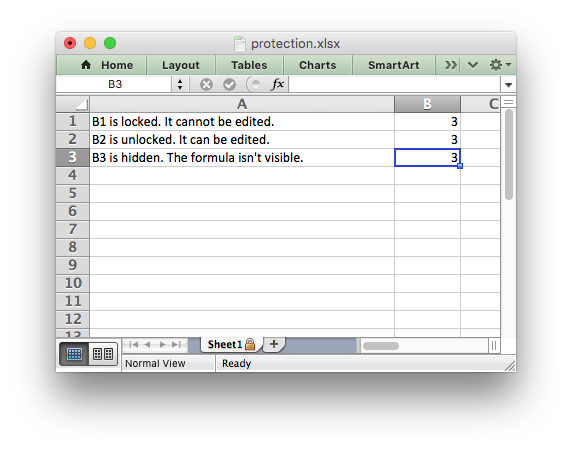libxlsxwriter
worksheet_protection.c

Example of setting Excel worksheet protection./*
* Example of cell locking and formula hiding in an Excel worksheet using
* libxlsxwriter.
*
* Copyright 2014-2021, John McNamara, jmcnamara@cpan.org
*
*/
#include "xlsxwriter.h"
int main() {
lxw_workbook *workbook = workbook_new("protection.xlsx");
/* Widen the first column to make the text clearer. */
worksheet_set_column(worksheet, 0, 0, 40, NULL);
/* Turn worksheet protection on without a password. */
worksheet_protect(worksheet, NULL, NULL);
/* Write a locked, unlocked and hidden cell. */
worksheet_write_string(worksheet, 0, 0, "B1 is locked. It cannot be edited.", NULL);
worksheet_write_string(worksheet, 1, 0, "B2 is unlocked. It can be edited.", NULL);
worksheet_write_string(worksheet, 2, 0, "B3 is hidden. The formula isn't visible.", NULL);
worksheet_write_formula(worksheet, 0, 1, "=1+2", NULL); /* Locked by default. */
worksheet_write_formula(worksheet, 1, 1, "=1+2", unlocked);
worksheet_write_formula(worksheet, 2, 1, "=1+2", hidden);
workbook_close(workbook);
return 0;
}
workbook_close
lxw_error workbook_close(lxw_workbook *workbook)
Close the Workbook object and write the XLSX file.
format_set_unlocked
void format_set_unlocked(lxw_format *format)
Set the cell unlocked state.
worksheet_write_formula
lxw_error worksheet_write_formula(lxw_worksheet *worksheet, lxw_row_t row, lxw_col_t col, const char *formula, lxw_format *format)
Write a formula to a worksheet cell.
workbook_new
lxw_workbook * workbook_new(const char *filename)
Create a new workbook object.
lxw_worksheet
Struct to represent an Excel worksheet.
Definition: worksheet.h:2107
lxw_format
Struct to represent the formatting properties of an Excel format.
Definition: format.h:358
worksheet_protect
void worksheet_protect(lxw_worksheet *worksheet, const char *password, lxw_protection *options)
Protect elements of a worksheet from modification.
lxw_workbook
Struct to represent an Excel workbook.
Definition: workbook.h:292
worksheet_write_string
lxw_error worksheet_write_string(lxw_worksheet *worksheet, lxw_row_t row, lxw_col_t col, const char *string, lxw_format *format)
Write a string to a worksheet cell.
worksheet_set_column
lxw_error worksheet_set_column(lxw_worksheet *worksheet, lxw_col_t first_col, lxw_col_t last_col, double width, lxw_format *format)
Set the properties for one or more columns of cells.